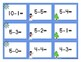# Winter Themed Hands On Subtraction Center FreebieSubject
Resource Type
File Type

PDF

(398 KB|8 pages)
Product Rating
Standards
Also included in:
1. These themed subtraction centers are hands on centers for students to practice subtracting numbers within 20. All subtraction facts from 20-20 down to 0-0 are included in this packet.What is included?A math mat for students to use manipulatives to solve math problemsA set of pumpkin themed math card
\$6.00
\$5.00
Save \$1.00
• Product Description
• StandardsNEW

This winter themed subtraction center is a hands on center for students to practice subtracting numbers within 20. Select subtraction facts from between 20-20 down to 0-0 are included in this packet.

Other Subtraction Centers

Pumpkin Themed Subtraction Centers

Add and subtract within 20, demonstrating fluency for addition and subtraction within 10. Use strategies such as counting on; making ten (e.g., 8 + 6 = 8 + 2 + 4 = 10 + 4 = 14); decomposing a number leading to a ten (e.g., 13 - 4 = 13 - 3 - 1 = 10 - 1 = 9); using the relationship between addition and subtraction (e.g., knowing that 8 + 4 = 12, one knows 12 - 8 = 4); and creating equivalent but easier or known sums (e.g., adding 6 + 7 by creating the known equivalent 6 + 6 + 1 = 12 + 1 = 13).
Fluently add and subtract within 5.
Solve addition and subtraction word problems, and add and subtract within 10, e.g., by using objects or drawings to represent the problem.
Represent addition and subtraction with objects, fingers, mental images, drawings, sounds (e.g., claps), acting out situations, verbal explanations, expressions, or equations.
Total Pages
8 pages
N/A
Teaching Duration
N/A
Report this Resource to TpT
Reported resources will be reviewed by our team. Report this resource to let us know if this resource violates TpT’s content guidelines.